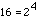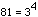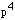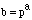Date: Thu, 17 Sep 1998 23:29:25 -0400 To whom it may concern, I have difficulty in getting the solution to the following question: Find 5 numbers that have exactly 5 factors. I got 16, 81 but couldn't find the rest. I believe that in order to have 5 factors, it has to be a square number. Isn't it true? I guess there may be a pattern to this. Thanks for your help. Derek Hi Derek 16 and 81 are squares, but they are also fourth powers.and. Notice also that 2 and 3 are primes. If p is a prime doeshave 5 factors? Since p is a prime, if b dividesthen b must be a power of p also, with its exponent at most 4. Thusand a is 0, 1, 2, 3 or 4. Hencehas 5 factors. Are primes to the fourth power the only numbers with exactly 5 factors? Cheers, Penny

Go to Math Central